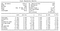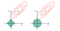Ro Data Sip-and-Share Q1 2019

Feature selection or feature pruning is a very crucial step in the pipeline of building a good prediction model and to understand the connections among the features and the target. The goal of feature selection is two-fold: 1. identify and remove features with little or no predictability of the target to prevent overfitting and 2. identify highly correlated or redundant features and suppress the negative impacts towards the model without losing critical information. In here, I will review the following approaches to achieve feature selection in the context of linear and logistic regression:

1. Statistical Inference
2. Greedy Search
3. Regularization

Statistical Inference

The statistical inference approach estimates the standard error the coefficients of regression model, and then constructs a confidence interval and p-value to test whether the coefficients are significantly different than 0. If the null hypothesis of the coefficient being zero is rejected with a small p-value, it means this feature has some genuine effects on the target.

The detailed derivation of the statistical inference of coefficients standard error can be find in this article for linear regression and this article for logistic regression. In here, I will only introduce the general concepts in this derivation and the practical steps to obtain the results. According to central limit theorem, the approximate distribution of the coefficients is a normal distribution for large sample size:

While the estimated coefficient approaches the true value β, the key to define this distribution is to estimate the standard deviation (σ) of the coefficient. This value can be thought of as a measure of the precision of the coefficient: if the standard error gets large comparing to the coefficient, the confidence interval gets wider and we’re less sure about where the true value lies.

The standard error of the model coefficients can be calculated as the square roots of the diagonal entries of the covariance matrix. In Python, the calculation for standard error of coefficients is available in statsmodels library, which also provides a series of statistical metrics that help make inference.Example of statsmodels’ logistic regression summary.

The statistical approach estimates the standard errors of the regression’s coefficients which serve as a direct metric to evaluate the connection between features and the target. However, removing features based on the output of significance test is not recommended because some features may not have significance in the statistical test but still possess certain prediction power towards the target, and removing those features often results in losing total information.

Greedy Search

Comparing to the statistical approach, the greedy search method is more practical and leaning towards the realm of machine learning engineering. The general idea of greedy search is to generate models with various combination of features and narrow down features subsets with the optimal model performance. There’re several alterations of greedy search strategy, and in here I will discuss two of them.

Univariate Selection

Univariate selection is the simplest approach among the greedy search methods. It evaluates how good a feature is by estimating its predictive value when taken alone in respect of the response and removes the features that perform poorly in the test. This method is best for the datasets that have large portion of redundant features (eg. Madelon dataset) as the initial pruning process.

Recursive Elimination

Recursive Elimination starts the feature selection process backwards with a full feature space. At each iteration, a random feature is removed and the performance of the model is reevaluated. If removing the feature has negligible effects on the model, then the feature can be safely pruned. This process stops when any further removal will hurt the predictability of the model.

Regularization

Regularization is another way of identifying and modifying important features to prevent overfitting, but without actively removing any features from the original dataset. To minimize the impact of meaningless and correlated features to the model, regularization curtails the coefficients of these features so that they do not contribute to the prediction results. This goal is achieved by adding penalization for the coefficients to the lost function.

The regularization dichotomizes into two separate branch based on how the coefficients are penalized. If the regularization penalizes on the absolute value of the coefficients (L1 norm), then the algorithm is referred as L1 regularization or Lasso regression. If the regularization penalizes on the sum of square of the coefficients (L2 norm), the algorithm is referred as L2 regularization or Ridge regression.

The little difference in the penalty term causes completely different behavior of these two regularization algorithms. Specifically, L1 regularization is able to assign feature coefficient to zero and therefore eliminating the entire impact of this feature while L2 regularization will assign a small coefficient to the insignificant feature.Demonstration of L1 (left) and L2 (right) regularization. (An Introduction to Statistical Learning by Gareth James, Daniela Witten, Trevor Hastie, Robert Tibshirani)

The diagram above is often used to illustrate why L1 regularization could have zero as coefficient in a 2-dimensional setup. The green area represents the coefficients’ operational region which shaped differently by the coefficient penalty term, and the red ellipses contour the convex of the original lost function. The optimal solution of the regularized regression is the first point at which the operational region intercepts with the convex. Lasso regression (on the left) has a diamond shaped constraint region with corners at each of the axes, which allows for intersecting the lost function at an axis, and the coefficient on the other axis equals to zero. On the contrary, Ridge regression (on the right) has a circular optional region with no sharp corners and this makes the intersection at an axis much less likely. For more explanation, please refer to this article.

In summary, L1 regularization, or the Lasso regression is often used as a method for feature selection due to its ability to assign zero as feature coefficients. Other variations of regularization including Least Angle Regression (LARS) and Elastic Net can be used for feature pruning as well, but all come with their own set of merits and limitations.

In here, I have reviewed some commonly used feature selection techniques which identify features that either have no predictable power towards the target or highly correlated with other features. There’re other ways to extract the information from the dataset, such as thresholding the feature variance and apply principle component analysis (PCA) to reduce dimensions in feature space. However, there’s no solution that can fit all the problems. More than often, the feature selection is a rather iterative process, and each step has unique pruning targets. Hence it’s important to understand how each algorithm fits in different scenario in order to achieve the ideal results.

Ro Data Team Blog

Ro Data Team Blog: data analytics, data engineering, data science

Welcome to a place where words matter. On Medium, smart voices and original ideas take center stage - with no ads in sight. Watch
Follow all the topics you care about, and we’ll deliver the best stories for you to your homepage and inbox. Explore
Get unlimited access to the best stories on Medium — and support writers while you’re at it. Just \$5/month. Upgrade